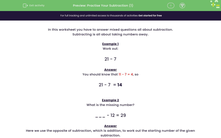# Practise Different Methods of Subtraction

In this worksheet, students will practise their subtraction skills.Key stage:  KS 2

Curriculum topic:   Number: Addition and Subtraction

Curriculum subtopic:   Solve Add/Subtract Problems to 1000

Popular topics:   Subtraction worksheets

Difficulty level:#### Worksheet Overview

In this activity, we will be answering mixed questions all about subtraction.

Subtracting is all about taking numbers away.

Example 1

Work out:

21 - 7

You probably know that 11 - 7 = 4, so

21 - 7  = 14

Example 2

What is the missing number?

___ - 12 = 29

Here, we use the opposite of subtraction, which is addition, to work out the starting number of the given subtraction.

We must work out 12 + 29.

Add the units 2 + 9 = 11

Add the tens 10 + 20 = 30

Add the two results 11 + 30 = 41

12 + 29 = 41

41 - 12 = 29Example 3

Work out:

43 - 29

Here we subtract 30 instead of 29.

Then we add 1 back at the end because we subtracted 1 too many by subtracting 30 instead of 29!

43 - 30 = 13

13 + 1 = 14

43 - 29  = 14

These are just a few tips to help you to work out subtraction problems in your head. Let's try putting them into practice in the questions that follow.### What is EdPlace?

We're your National Curriculum aligned online education content provider helping each child succeed in English, maths and science from year 1 to GCSE. With an EdPlace account you’ll be able to track and measure progress, helping each child achieve their best. We build confidence and attainment by personalising each child’s learning at a level that suits them.

Get started